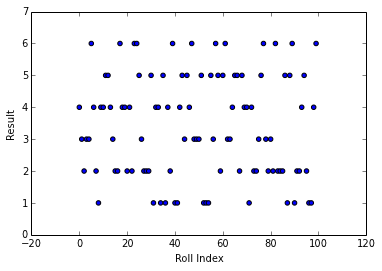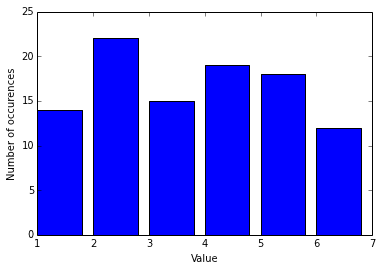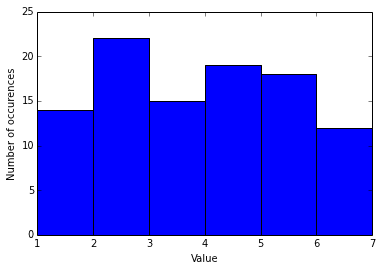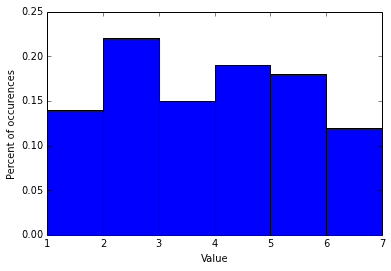# Uniform Random Probabilities¶

## If I roll a die 100 times, how many times will it come up a 6?¶

In :
import random
import math

num_rand_vals = 100
min_value = 1
max_value = 6

rand_vals = []

print "Roll: number"
for i in xrange(num_rand_vals):
val = random.randint(min_value, max_value)
rand_vals.append(val)
if (i < 10):
print "%d: %d" % (i, val)

Roll: number
0: 4
1: 3
2: 2
3: 3
4: 3
5: 6
6: 4
7: 2
8: 1
9: 4

In :
min_seen = min(rand_vals)
max_seen = max(rand_vals)
mean_seen = (sum(rand_vals)+0.0) / len(rand_vals)

print "When simulating %d random values between %d and %d" % (num_rand_vals, min_value, max_value)
print "The smallest I saw was %d" % (min_seen)
print "The biggest I saw was %d" % (max_seen)
print "And the mean value was %.02f" % (mean_seen)

When simulating 100 random values between 1 and 6
The smallest I saw was 1
The biggest I saw was 6
And the mean value was 3.41

In :
## Plot the individual vals
plt.figure()
plt.xlabel("Roll Index")
plt.ylabel("Result")
plt.scatter(range(num_rand_vals), rand_vals)
plt.show()In :
## summarize how many times we saw each value
tally = [0 for x in xrange(max_value+1)]
for i in rand_vals:
tally[i] += 1

print "value #times_seen"
for t in xrange(len(tally)):
print "%d: %d" % (t, tally[t])

value #times_seen
0: 0
1: 14
2: 22
3: 15
4: 19
5: 18
6: 12


## Why does 0 have 0?¶

In :
print "We expected to see the number 6 %.02f percent of the time = %d times" % (100./6, num_rand_vals/6)
print "We actually saw 6 %d times" % (tally)

We expected to see the number 6 16.67 percent of the time = 16 times
We actually saw 6 12 times

In :
## summarize the tally
mean_tally = (sum(tally) + 0.) / (len(tally)-1) ## skip 0
min_tally = tally

sumdiff = 0.0
for i in xrange(min_value, max_value+1):
diff = (tally[i] - mean_tally) ** 2
sumdiff += diff
if (tally[i] < min_tally):
min_tally = tally[i]

sumdiff /= (len(tally)-1)
stdev_tally = math.sqrt(sumdiff)

print "the minimum tally was %d" % (min_tally)
print "the maximum tally was %d" % (max(tally))
print "the mean tally was %.02f +/- %.2f" %  (mean_tally, stdev_tally)

the minimum tally was 12
the maximum tally was 22
the mean tally was 16.67 +/- 3.35

In :
## plot the tally, a histogram
plt.figure()
plt.xlabel("Value")
plt.ylabel("Number of occurences")
plt.bar(range(max_value+1), tally)
plt.show()In :
## The hist function will do everything for us in one step
plt.figure()
plt.xlabel("Value")
plt.ylabel("Number of occurences")
plt.hist(rand_vals, bins=range(max_value+2))
plt.show()In :
## The hist function can also plot the probability of each number, i.e. the density function
plt.figure()
plt.xlabel("Value")
plt.ylabel("Percent of occurences")
plt.hist(rand_vals, bins=range(max_value+2), normed=True)
plt.show()